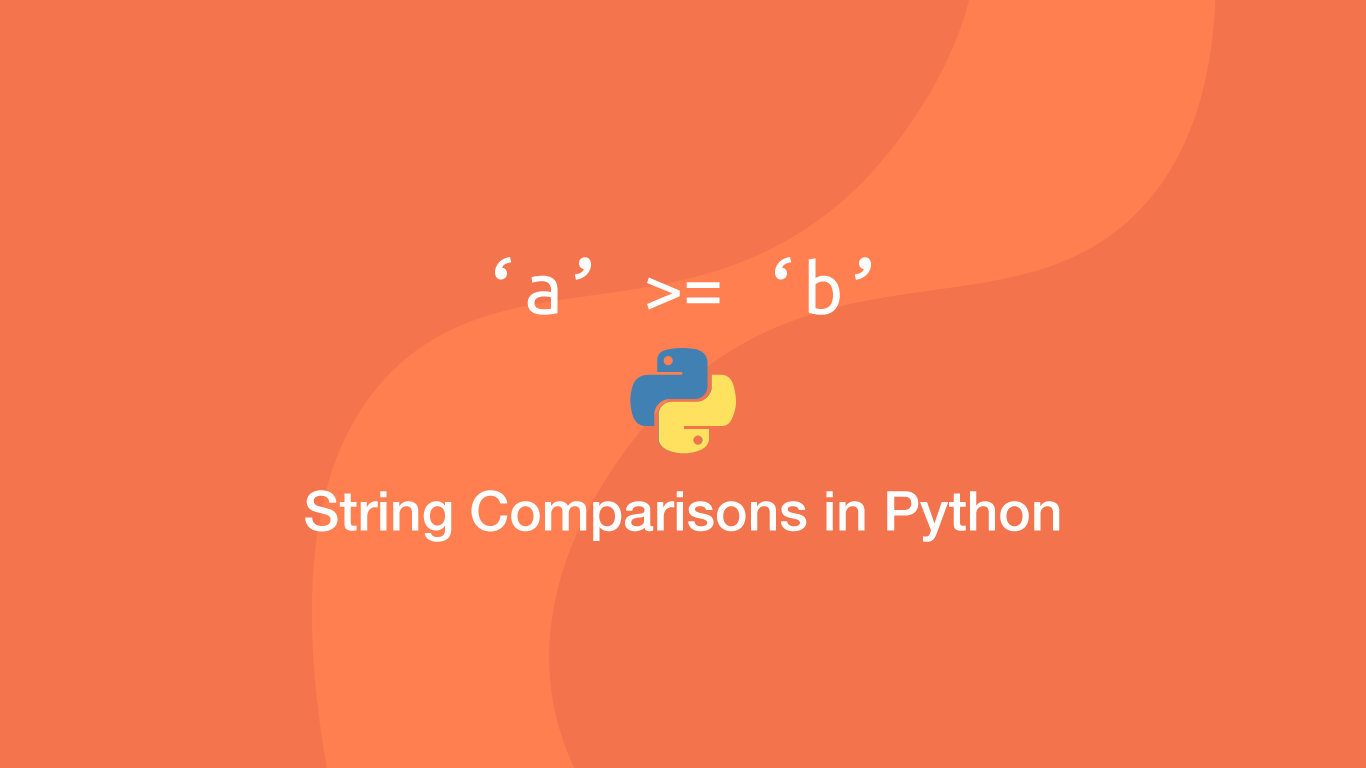# How to Compare Strings in PythonA string in Python is an iterable data type with each character in the string having its own index. As a result, it is possible to compare strings in a variety of different ways such as comparing lengths or comparing individual indexes on each string.

In this tutorial, we will learn how to compare strings in Python with examples.

## How String Comparisons Work in Python

Python compares strings on a character by character basis. Characters are ordered alphabetically with the last letter `z` having the highest value and `a` the lowest; capital letters are less than lowercase letters and numbers are less than all letters. Here are a couple of examples to demonstrate how this works:

`a` is not greater than `b`

``````result = 'a' > 'b'
print(result)
``````
``````False
``````

`a` is greater than `A`

``````result = 'a' > 'A'
print(result)
``````
``````True
``````

`a` is greater than `5`

``````result = 'a' > '5'
print(result)
``````
``````True
``````

Knowing how string comparisons are performed by Python it is easy to see why the statement below returns `True`.

``````result = 'abdd' > 'abcd'
print(result)
``````
``````True
``````

Python iterates through each character and when it sees that `d` is greater than `c` `True` is returned.

## The Result of a String Comparison

A comparison statement will always return `True` or `False`. `True` meaning correct, a match was found and `False` for no match. This means the result can be stored in a variable or used directly on `if` logic.

``````result = 'a' == 'a'

if (result):
print(result)
``````
``````True
``````

## The Python Comparison Operators

Here are the Python operators that can be used to make string comparisons:

• `>` - greater than
• `<` - less than
• `==` - equal to; not to be confused with `=` (variable assignment)
• `>=` - greater than or equal to
• `<=` - less than or equal to
• `!=` - not equal to

Here are a couple of examples of using different comparison operators:

``````# not equal to
result = 'a' != 'b'

print(result)
``````
``````True
``````
``````# less than or equal to
result = 'a' <= 'b'

print(result)
``````
``````True
``````
``````# greater than or equal to
result = 'a' >= 'b'

print(result)
``````
``````False
``````

## Compare Strings and Number Objects

Python does not automatically convert number objects into strings when compared with a string. To compare strings and numbers, the number will need to be converted to a string using str().

``````result = '1' == 1

print(result)
``````
``````False
``````
``````result = '1' == str(1)

print(result)
``````
``````True
``````

## Case-Insensitive Comparisons

Since characters are compared lexicographically, strings with different cases will have to be converted to lower or upper case using the `upper()` or `lower()` methods for them to return `True`.

``````str_1 = 'Manchester'
str_2 = 'MANCHESTER'

result = str_1.lower() == str_2.lower()

print(result)
``````
``````True
``````

## Check if a String is Longer than Another String

To see if one string is longer than another we get the length of each string using the `len()` function and compare the output using a `>` (greater than) comparison statement.

``````str_1 = 'Manchester'
str_2 = 'MANCHESTER'

result = len(str_1) > len(str_2)

print(result)
``````
``````False
``````

## Match Strings with Regular Expressions

To match strings with regex, import the native `re` Python package. Then a regex pattern can be stored in a variable using the `re.compile()` method.

``````import re

str_list = ['apple', 'two', 'too']

pattern = re.compile('t[wo]o')

for s in str_list:
if pattern.search(s):
print('Match!', s)
else:
print('No match:', s)
``````
``````No match: apple
Match! two
Match! too
``````

The regex in the above example is `[]` (square brackets) to match any one of the two characters contained within the brackets.

## Compare Multi-line Strings

To compare multi-line strings in Python we can use the `difflib` package. Once `difflib` has been imported, use the `difflib.Differ()` to create a new `difflib` object and then use the `compare()` method, passing the strings to compare as two arguments.

``````import difflib

string = """hello this is a line
and this is a line
and another line"""

string_edit = """hello this is a line
and this is an updated line
and another line"""

diff = difflib.Differ()

result = diff.compare(string.split('\n'), string_edit.split('\n'))

print('\n'.join(result))
``````
``````  hello this is a line
- and this is a line
+ and this is an updated line
?              +++++++++

and another line
``````

`difflib` will return lines starting with a `-` (dash) that have been changed followed by the updated line starting with a `+` (plus).

## Conclusion

You now know how to work with strings in Python, including the available comparison statements and how Python evaluates strings.

#### Related Tutorials### How to Compare Values in JavaScript

August 26, 2020### How to use Python datetime to Create and use Dates and Times

September 24, 2020### PHP DateTime - Create, Format and Compare Dates with PHP

September 04, 2020### How to Calculate Mean, Median, Mode and Range in Python

October 07, 2020### How to use the XOR operator in Python

April 07, 2021June 23, 2021